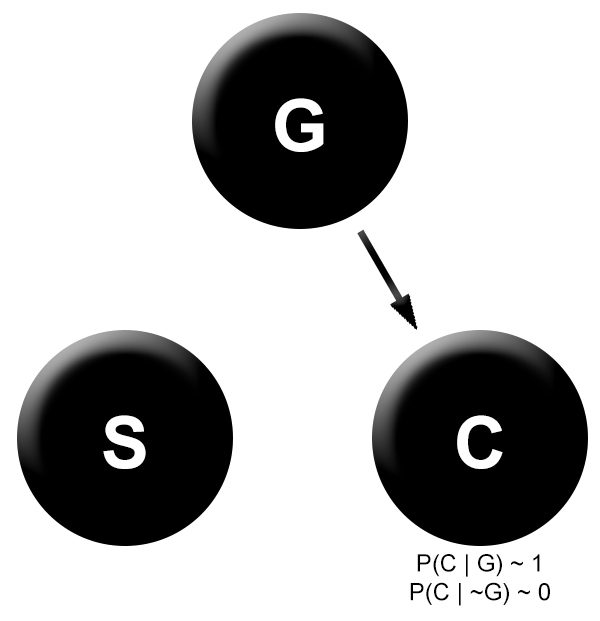# Causal decision theory

We’ll now move on into slightly new intellectual territory, that of decision theory.

While what we’ve previously discussed all had to do with questions about the probabilities of events and causal relationships between variables, we will now discuss questions about what the best decision to make in a given context is.

***

Decision theory has two ingredients. The first is a probabilistic model of different possible events that allows an agent to answer questions like “What is the probability that A happens if I do B?” This is, roughly speaking, the agent’s beliefs about the world.

The second ingredient is a utility function U over possible states of the world. This function takes in propositions, and returns the value to a particular agent of that proposition being true. This represents the agent’s values.

So, for instance, if A = “I win a million dollars” and B = “Somebody cuts my ear off”, U(A) will be a large positive number, and U(B) will be a large negative number. For propositions that an agent feels neutral or apathetic about, the utility function assigns them a value of 0.

Different decision theories represent different ways of combining a utility function with a probability distribution over world states. Said more intuitively, decision theories are prescriptions for combining your beliefs and your values in order to yield decisions.

A proposition that all competing decision theories agree on is “You should act to maximize your expected utility.” The difference between these different theories, then, is how they think that expected utility should be calculated.

“But this is simple!” you might think. “Simply sum over the value of each consequence, and weight each by its likelihood given a particular action! This will be the expected utility of that action.”

This prescription can be written out as follows:Here A is an action, C is the index for the different possible world states that you could end up in, and K is the conjunction of all of your background knowledge.

***

While this is quite intuitive, it runs into problems. For instance, suppose that scientists discover a gene G that causes both a greater chance of smoking (S) and a greater chance of developing cancer (C). In addition, suppose that smoking is known to not cause cancer.The question is, if you slightly prefer to smoke, then should you do so?

The most common response is that yes, you should do so. Either you have the cancer-causing gene or you don’t. If you do have the gene, then you’re already likely to develop cancer, and smoking won’t do anything to increase that chance.

And if you don’t have the gene, then you already probably won’t develop cancer, and smoking again doesn’t make it any more likely. So regardless of if you have the gene or not, smoking does not affect your chances of getting cancer. All it does is give you the little utility boost of getting to smoke.

But our expected utility formula given above disagrees. It sees that you are almost certain to get cancer if you smoke, and almost certain not to if you don’t. And this means that the expected utility of smoking includes the utility of cancer, which we’ll suppose to be massively negative.

Let’s do the calculation explicitly:

EU(S) = U(C & S) * P(C | S) + U(~C & S) * P(~C| S)
= U(C & S) << 0
EU(~S) =  U(~S & C) * P(C | ~S) + U(~S & ~C) * P(~C | ~S)
= U(~S & ~C) ~ 0

Therefore we find that EU(~S) >> EU(S), so our expected utility formula will tell us to avoid smoking.

The problem here is evidently that the expected utility function is taking into account not just the causal effects of your actions, but the spurious correlations as well.

The standard way that decision theory deals with this is to modify the expected utility function, switching from ordinary conditional probabilities to causal conditional probabilities.You can calculate these causal conditional probabilities by intervening on S, which corresponds to removing all its incoming arrows.Now our expected utility function exactly mirrors our earlier argument – whether or not we smoke has no impact on our chance of getting cancer, so we might as well smoke.

Calculating this explicitly:

EU(S) = U(S & C) * P(C | do S) + U(S & ~C) * P(~C | do S)
= U(S & C) * P(C) + U(S & ~C) * P(~C)
EU(~S) = U(~S & C) * P(C | do ~S) + U(S & ~C) * P(~C | do S)
= U(~S & C) * P(C) + U(~S & ~C) * P(~C)

Looking closely at these values, we can see that EU(S) must be greater than EU(~S), regardless of the value of P(C).

***

The first expected utility formula that we wrote down represents the branch of decision theory called evidential decision theory. The second is what is called causal decision theory.

We can roughly describe the difference between them as that evidential decision theory looks at possible consequences of your decisions as if making an external observation of your decisions, while causal decision theory looks at the consequences of your decisions as if determining your decisions.

EDT treats your decisions as just another event out in the world, while CDT treats your decisions like causal interventions.

Perhaps you think that the choice between these is obvious. But Newcomb’s problem is a famous thought experiment that famously splits people along these lines and challenges both theories. I’ve written about it here, but for now will leave decision theory for new topics.